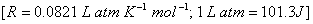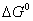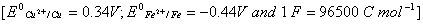Tuesday 29th September 2020

CBSE Guess > Papers > Question Papers > Class XII > 2003 > Chemistry > Compartment Outside Delhi Set -II.

CHEMISTRY—2003 (Set II—Compartment Outside Delhi)

Note: Except for the following questions, all the remaining questions, all the remaining questions have been asked in Set I .

Q. 1. Give an example of a solution containing a solid solute in a solid solvent. 1

Q. 5. What is the effect of Frenkel defect on electrical conductivity of the solid? 1

Q. 8. Which quantum number will express the difference in two electrons in K-hell? 1

Q. 12. A 5 litre cylinder contained 10 moles of oxygen gas at 27 0C. Due to sudden leakage through the hole, all the gas escaped into the atmosphere and the cylinder got empty. If the atmospheric pressure is 1,0 atm, calculate the work done by the gas.Q. 14. The rate constant for a first order rection becomes six times when the temperature is raised from 350 K to 400 K. Calculate the activation energy for the reaction. 2Q. 15. Calculate the standard electrode potential of Ni2+ | Ni electrode if the cell potential of the cell 2Q. 24. Blue colour of copper sulphate solution is slowly discharged (disappears) when an iron- rod is dipped into it Explain this bywith the help of the following data: 2Q. 27. How are organometallic compounds classified Draw the structure of Zeise's salt. State two important applications of coordination complexes in qualitative analysis of cations. 3

Q. 29. (a) Name any two projectiles commonly used in artifical transmutation of elements.
(b) Half-life period of a radioactive element is 100 seconds. Calculate the disintegration constant. How much time will the element take for 90% of its decay?

Q. 31. Describe the following: 3
(i) Functions of carbohydrates in plants
(ii) Replication
(iii) Protein synthesis of nucleic acids

 Chemistry 2003 Question Papers Class XII Delhi Outside Delhi Compartment Delhi Compartment Outside DelhiSet ISet ISet ISet ISet IISet IISet IISet II

CBSE 2003 Question Papers Class XII